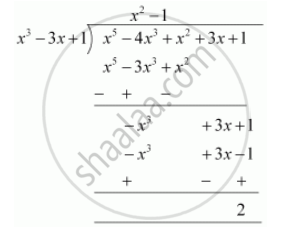Share
Notifications

View all notifications
Books Shortlist
Your shortlist is empty

# Check whether the first polynomial is a factor of the second polynomial by dividing the second polynomial by the first polynomial x^3 – 3x + 1, x^5 – 4x^3 + x^2 + 3x + 1 - CBSE Class 10 - Mathematics

Login
Create free account

Forgot password?
ConceptDivision Algorithm for Polynomials

#### Questions

Check whether the first polynomial is a factor of the second polynomial by dividing the second polynomial by the first polynomial

x3 – 3x + 1, x5 – 4x3 + x2 + 3x + 1

Check whether the first polynomial is a factor of the second polynomial by applying the division algorithm g(x) = x3 – 3x + 1, f(x) = x5 – 4x3 + x2 + 3x + 1

#### Solution

x3 - 3x + 1, x5 - 4x3 + x2 + 3x + 1Since the remainder ≠ 0

Hence x3 - 3x + 1 is not a factor of x5 - 4x3 + x2 + 3x + 1

Is there an error in this question or solution?

#### APPEARS IN

NCERT Solution for Mathematics Textbook for Class 10 (2019 to Current)
Chapter 2: Polynomials
Ex.2.30 | Q: 2.3 | Page no. 36
RD Sharma Solution for 10 Mathematics (2018 to Current)
Chapter 2: Polynomials
Ex.2.30 | Q: 2.2 | Page no. 57
NCERT Solution for Mathematics Textbook for Class 10 (2018 to Current)
Chapter 2: Polynomials
Ex.2.30 | Q: 2.3 | Page no. 36

#### Video TutorialsVIEW ALL 

Solution Check whether the first polynomial is a factor of the second polynomial by dividing the second polynomial by the first polynomial x^3 – 3x + 1, x^5 – 4x^3 + x^2 + 3x + 1 Concept: Division Algorithm for Polynomials.
S